## LetsPlayMaths.com

WELCOME TO THE WORLD OF MATHEMATICS

Addition of 2-Digit and 1-Digit Numbers

Story Sum

Addition means keeping things together. For addition we put ‘+’ sign, and it is known as ADD/PLUS sign.

Example 1. Add the below given red and blue balls.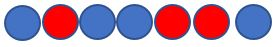Solution.We have 4 Blue balls and 3 red balls.
Total number of balls = 4 + 3 = 7 balls
So, we have total 7 blue and red balls

Example 2. Add the total number of White and green boxes.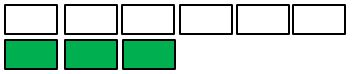Solution. We have 6 white boxes and 3 green boxes.
Total number of boxes = 6 + 3 = 9 boxes
So, we have total 9 white and green boxes.

During the addition, if we change the order of numbers then the answer does not change.
Addition of 3 yellow balls and 4 green balls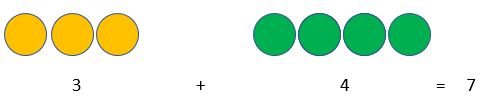Addition of 4 green balls and 3 yellow balls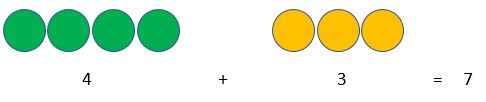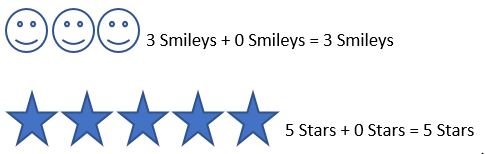When we add 1 to a number, the answer is the successor number.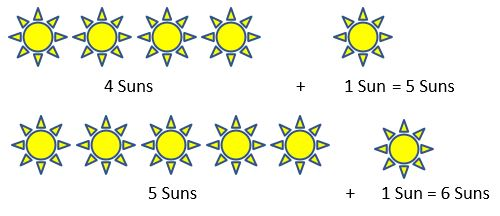John is having 3 smileys, Bob is having 5 smileys and Julie is having 2 smileys. How many total smileys they have with them?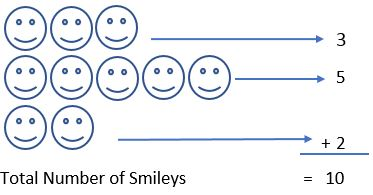So, in total they have 10 smileys with them.

## Addition of 2-Digit and 1-Digit Numbers## Story Sum

In our day to day life we will come across with these simple additions.

Example 1. Ram has 5 chocolates and Sita has 4 chocolates. What is the total number of chocolates they both have?
Solution.
Number of chocolates Ram has = 5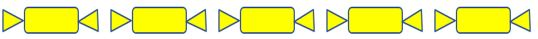Number of chocolate Sita has = 4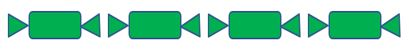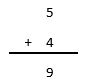So, Ram and Sita both have 9 chocolates.